Python 电信用户流失预测模型实战教程-Python 实用宝典

# Python 电信用户流失预测模型实战教程

## 1.流失预测 研究背景

1、做好`用户流失预测`可以降低营销成本。老生常谈，`新客户开发成本``老客户维护成本`的5倍。

2、获得更好的用户体验。并不是所有的增值服务都可以有效留住客户。

3、获得更高的销售回报。价格敏感型客户和非价格敏感性客户。

## 2.提出问题

1、流失客户有哪些显著性特征？

2、当客户在哪些特征下什么条件下比较容易发生流失

## 3.数据集描述

https://www.datafountain.cn/datasets/35guide

`customerID` ：用户ID
`gender`：性别（Female & Male）
`SeniorCitizen` ：老年人（1表示是，0表示不是）
`Partner` ：是否有配偶（Yes or No）
`Dependents` ：是否经济独立（Yes or No）
`tenure` ：客户的职位（0-72，共73个职位）

`PhoneService` ：是否开通电话服务业务（Yes or No）
`MultipleLines` ：是否开通了多线业务（Yes 、No or No phoneservice 三种）
`InternetService` ：是否开通互联网服务（No, DSL数字网络，fiber optic光纤网络 三种）
`OnlineSecurity` ：是否开通网络安全服务（Yes，No，No internetserive 三种）
`OnlineBackup` ：是否开通在线备份业务（Yes，No，No internetserive 三种）
`DeviceProtection` ：是否开通了设备保护业务（Yes，No，No internetserive 三种）
`TechSupport` ：是否开通了技术支持服务（Yes，No，No internetserive 三种）
`StreamingTV` ：是否开通网络电视（Yes，No，No internetserive 三种）
`StreamingMovies` ：是否开通网络电影（Yes，No，No internetserive 三种）
`Contract` ：签订合同方式 （按月，一年，两年）
`PaperlessBilling` ：是否开通电子账单（Yes or No）
`PaymentMethod` ：付款方式（bank transfer，credit card，electronic check，mailed check）
`MonthlyCharges` ：月费用
`TotalCharges` ：总费用

## 4.分析思路

`分析视角``分析方法`的灵魂。

• 对比视角
• 分类视角
• 相关视角
• 描述视角

• 哪些属性的用户比较容易流失？
• 哪些行为的用户比较容易流失？

• 数值型数据：均值比较
• 分类型数据：频数分布比较（交叉分析）

`权重问题`属于`分类视角`，故我们可以采用`分类模型`，要用哪个`分类模型`呢？不知道。可以全部采用，看模型精度得分，然后选得分最高的模型进行进一步预测。

• Random Forest 随机森林
• SVC 支持向量机
• LogisticRegression 逻辑回归
• KNN 近邻算法
• Naive Bayes  朴素贝叶斯
• Decision Tree 决策树
• XGB
• CatBoost

## 5.分析结论及运营建议

### 5.1 分析结论

1. tenure ：1-5号职位的用户比较容易流失
2. PaymentMethod ：使用`电子支票`支付的人
3. MonthlyCharges 、TotalCharges : 总费用在2281.92元以下，月费用在64.76元以上的客户比较容易流失
4. PaperlessBilling : 开通电子账单
5. Partner : 单身
6. OnlineBackup : 没开通`在线备份业务`
7. InternetService ：开通了`Fiber optic 光纤网络`
8. TechSupport ：没开通“技术支持服务”
9. DeviceProtection ：没开通`设备保护业务`
10. OnlineSecurity ：没开通`网络安全服务`
11. Contract ：`按月`签订合同方式
12. Dependents ：无经济独立
13. SeniorCitizen ：青年人
14. TotalCharges ：总费用在2281.92元以下，月费用在64.76元以上的客户比较容易流失

### 5.2 运营建议

• 增加用户的沉没成本（损失厌恶）
• 会员等级
• 积分制
• 充值赠送
• 满减券
• 其他增值服务
• 培养用户的条件反射（习惯）
• 会员日
• 定期用户召回
• 签到
• 每日定时抽奖
• 小游戏

### 电子账单解锁新权益

• `现象`：“开通电子账单”的人反而容易流失。
• `基本假设`：价格敏感型客户。电子账单，让客户理性消费。
• `建议`：让“电子账单”变成一项“福利。跟连锁便利店，联名发"商品满减券"，每月的账单时间，就将"商品满减券“和账单一起推送过去。文案：您上月消费了XX元，解锁了xx会员权益。
• `底层规律`：增加沉没成本。

### “单身用户”尊享亲情网

• `现象`：“单身用户”容易流失。
• `基本假设`：社交欲望低。
• `建议`：一个单身用户拥有建立3个人以内的“亲情网”的权益。
• `底层规律`：增加沉没成本。

## 6.实战教程-数据清洗

### 6.1 导入模块

#### 6.1.1 数据处理

``import pandas as pdimport numpy as np``

#### 6.1.2 可视化

``import matplotlib.pyplot as pltimport seaborn as snssns.set(style='darkgrid',font_scale=1.3)plt.rcParams['font.family']='SimHei'plt.rcParams['axes.unicode_minus']=False``

### 6.1.3 特征工程

``import sklearnfrom sklearn import preprocessing                            #数据预处理模块from sklearn.preprocessing import LabelEncoder               #编码转换from sklearn.preprocessing import StandardScaler             #归一化from sklearn.model_selection import StratifiedShuffleSplit   #分层抽样from sklearn.model_selection import train_test_split         #数据分区from sklearn.decomposition import PCA                        #主成分分析 （降维）``

#### 6.1.4 分类算法

``from sklearn.ensemble import RandomForestClassifier     #随机森林from sklearn.svm import SVC,LinearSVC                   #支持向量机from sklearn.linear_model import LogisticRegression     #逻辑回归from sklearn.neighbors import KNeighborsClassifier      #KNN算法from sklearn.cluster import KMeans                     #K-Means 聚类算法from sklearn.naive_bayes import GaussianNB              #朴素贝叶斯from sklearn.tree import DecisionTreeClassifier         #决策树``

#### 6.1.5 分类算法--集成学习

``import xgboost as xgbfrom xgboost import XGBClassifier                      from catboost import CatBoostClassifier                from sklearn.ensemble import AdaBoostClassifier        from sklearn.ensemble import GradientBoostingClassifier ``

#### 6.1.6 模型评估

``from sklearn.metrics import classification_report,precision_score,recall_score,f1_score  #分类报告from sklearn.metrics import confusion_matrix           #混淆矩阵from sklearn.metrics import silhouette_score           #轮廓系数（评价k-mean聚类效果）from sklearn.model_selection import GridSearchCV       #交叉验证from sklearn.metrics import make_scorerfrom sklearn.ensemble import VotingClassifier          #投票``

#### 6.1.7 忽略警告

``import warningswarnings.filterwarnings('ignore')``

### 6.2 读取数据

``df=pd.read_csv(r'C:\Users\Think\Desktop\刻意练习数据\电信数据集\Customer-Churn.csv',header=0)#预览数据df.head()#查看数据大小df.shape#查看数据数据及分布df.describe()``

### 6.3 数据清洗

#### 6.3.1 缺失值处理

``#查看缺失值df.isnull().sum()``

#### 6.3.2 重复值处理

``#查看重复值df.duplicated().sum()``

【输出】#### 6.3.3 数值类型转换

``#查看数据类型df.info()``

【输出】`TotalCharages`总费用应该跟`MonthlvCharges`是同一个数据类型(float64)。故需将TotalCharages由object转换成float64，且需要再次查看缺失值。

``#总费用 TotalCharges  该列的数据类型应是float64，不是object# df['TotalCharges'].astype('float64')# 此处用“astype”转化数据类型报错 “could not convert string to float”#改用强制转化 convert_numeric=True   df['TotalCharges']=df['TotalCharges'].convert_objects(convert_numeric=True)df['TotalCharges'].dtype``

• 分类型数据：众数填充
• 数值型数据：正态分布，均值/中位数填充；偏态分布，中位数填充。

TotalCharges列是数值型数据，先画直方图查看数据分布形态。

``#分别作直方图：全部客户类型、流失客户类型、留存客户类型plt.figure(figsize=(14,5))plt.subplot(1,3,1)plt.title('全部客户的总付费直方图')sns.distplot(df['TotalCharges'].dropna())plt.subplot(1,3,2)plt.title('流失客户的总付费直方图')sns.distplot(df[df['Churn']=='Yes']['TotalCharges'].dropna())plt.subplot(1,3,3)plt.title('留存客户的总付费直方图')sns.distplot(df[df['Churn']=='No']['TotalCharges'].dropna())``

``df.fillna({'TotalCharges':df['TotalCharges'].median()},inplace=True)#再次确认是否还有空值df.isnull().sum()``

### 6.4 查看样本分布

``df['Churn'].replace(to_replace = 'Yes', value = 1,inplace = True)df['Churn'].replace(to_replace = 'No', value = 0,inplace = True)``

``df['Churn']=df['Churn'].map({'Yes':1,'No':0})``

``df['Churn'].head()``

``churn_value=df["Churn"].value_counts()labels=df["Churn"].value_counts().indexplt.figure(figsize=(7,7))plt.pie(churn_value,labels=labels,colors=["b","w"], explode=(0.1,0),autopct='%1.1f%%', shadow=True)plt.title("流失客户占比高达26.5%")plt.show()  ``

• 分层抽样
• 过抽样
• 欠抽样

## 7.实战教程-特征选择

``feature=df.iloc[:,1:20]``

### 7.1 整数编码

``#重新编码corr_df = feature.apply(lambda x: pd.factorize(x))corr_df.head()#相关性矩阵corr=corr_df.corr()corr``

``#绘制热力图观察变量之间的相关性强弱plt.figure(figsize=(15,12))ax = sns.heatmap(corr, xticklabels=corr.columns, yticklabels=corr.columns,                  linewidths=0.2, cmap="RdYlGn",annot=True)plt.title("Correlation between variables")``

### 7.2 独热编码

``df_onehot = pd.get_dummies(df.iloc[:,1:21])df_onehot.head()``

``plt.figure(figsize=(15,6))df_onehot.corr()['Churn'].sort_values(ascending=False).plot(kind='bar')plt.title('Correlation between Churn  and variables ')``

``kf_var=list(df.columns[2:5])for var in list(df.columns[7:18]):    kf_var.append(var)print('kf_var=',kf_var)``

## 8.实战教程-统计分析

### 8.1 频数分布比较

#### 8.1.1 卡方检验

"卡方检验",就是提高频数比较结论可信度的统计方法。

``#分组间确实是有显著性差异，频数比较的结论才有可信度，故需进行”卡方检验“from scipy.stats import chi2_contingency   #统计分析 卡方检验#自定义卡方检验函数def KF(x):    df1=pd.crosstab(df['Churn']，df[x])    li1=list(df1.iloc[0,:])    li2=list(df1.iloc[1,:])    kf_data=np.array([li1,li2])    kf=chi2_contingency(kf_data)    if kf<0.05:        print('Churn by {} 的卡方临界值是{:.2f}，小于0.05，表明{}组间有显著性差异,可进行【交叉分析】'.format(x,kf,x),'\n')    else:        print('Churn by {} 的卡方临界值是{:.2f}，大于0.05，表明{}组间无显著性差异,不可进行交叉分析'.format(x,kf,x),'\n')#对 kf_var进行卡方检验print('kf_var的卡方检验结果如下:','\n')print(list(map(KF, kf_var)))``

kf_var的卡方检验结果如下:

Churn by SeniorCitizen 的卡方临界值是0.00，小于0.05，表明SeniorCitizen组间有显著性差异,可进行【交叉分析】

Churn by Partner 的卡方临界值是0.00，小于0.05，表明Partner组间有显著性差异,可进行【交叉分析】

Churn by Dependents 的卡方临界值是0.00，小于0.05，表明Dependents组间有显著性差异,可进行【交叉分析】

Churn by MultipleLines 的卡方临界值是0.99，大于0.05，表明MultipleLines组间无显著性差异,不可进行交叉分析

Churn by InternetService 的卡方临界值是0.00，小于0.05，表明InternetService组间有显著性差异,可进行【交叉分析】

Churn by OnlineSecurity 的卡方临界值是0.00，小于0.05，表明OnlineSecurity组间有显著性差异,可进行【交叉分析】

Churn by OnlineBackup 的卡方临界值是0.00，小于0.05，表明OnlineBackup组间有显著性差异,可进行【交叉分析】

Churn by DeviceProtection 的卡方临界值是0.00，小于0.05，表明DeviceProtection组间有显著性差异,可进行【交叉分析】

Churn by TechSupport 的卡方临界值是0.00，小于0.05，表明TechSupport组间有显著性差异,可进行【交叉分析】

Churn by StreamingTV 的卡方临界值是0.00，小于0.05，表明StreamingTV组间有显著性差异,可进行【交叉分析】

Churn by StreamingMovies 的卡方临界值是0.00，小于0.05，表明StreamingMovies组间有显著性差异,可进行【交叉分析】

Churn by Contract 的卡方临界值是0.00，小于0.05，表明Contract组间有显著性差异,可进行【交叉分析】

Churn by PaperlessBilling 的卡方临界值是0.00，小于0.05，表明PaperlessBilling组间有显著性差异,可进行【交叉分析】

Churn by PaymentMethod 的卡方临界值是0.00，小于0.05，表明PaymentMethod组间有显著性差异,可进行【交叉分析】

#### 8.1.2 柱形图

``plt.figure(figsize=(20,25))a=0for k in kf_var:    a=a+1    plt.subplot(4,4,a)    plt.title('Churn BY '+ k)    sns.countplot(x=k,hue='Churn',data=df)``

``plt.xticks(rotation=45)sns.countplot(x='PaymentMethod',hue='Churn',data=df)``可以直接从柱形图去判断对哪个维度对流失客户的影响大吗？不能，因为“样本不均衡”（流失客户样本占比26.5%，留存客户样本占比73.5%），基数不一样，故不能直接通过“频数”的柱形图去分析。

#### 8.1.3 交叉分析

``print('ka_var列表中的维度与Churn交叉分析结果如下：','\n')for i in kf_var:    print('................Churn BY {}...............'.format(i))    print(pd.crosstab(df['Churn'],df[i],normalize=0),'\n') #交叉分析，同行百分比``

ka_var列表中的维度与Churn交叉分析结果如下：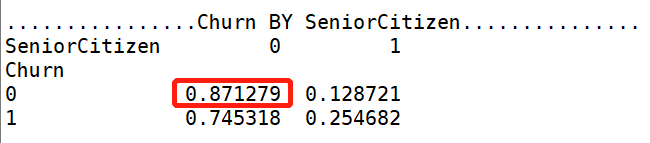【SeniorCitizen 分析】：年轻用户 在流失、留存，两个标签的人数占比都高。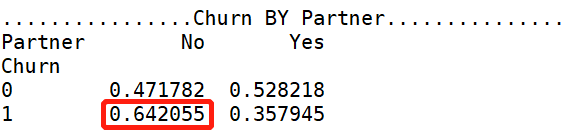【Parter 分析】：单身用户更容易流失。【Denpendents 分析】：经济不独立的用户更容易流失。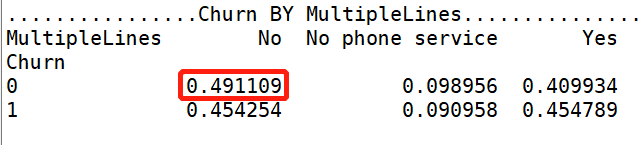【MultipleLines 分析】：是否开通MultipleLines，对留存和流失都没有明显的促进作用。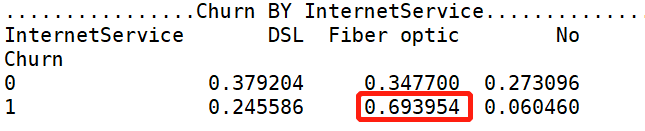【InternetService 分析】：办理了 “Fiber optic 光纤网络”的客户容易流失。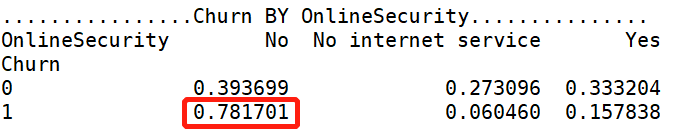【OnlineSecurity 分析】：没开通“网络安全服务”的客户容易流失。【OnlineBackup 分析】：没开通“在线备份服务”的客户容易流失。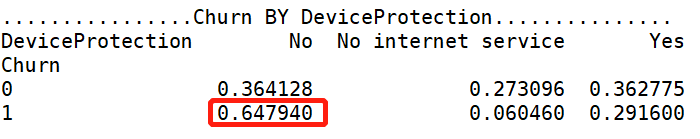【DeviceProtection 分析】：没开通“设备保护业务”的用户比较容易流失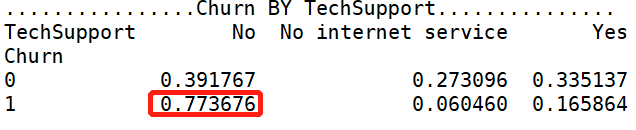【TechSupport 分析】：没开通“技术支持服务”的用户容易流失。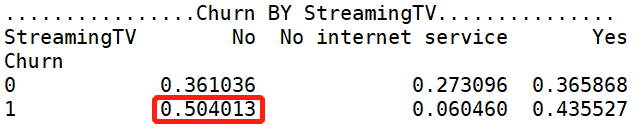【StreamingTV 分析】：是否开通“网络电视”服务，对用户留存、流失，没有明显的促进作用。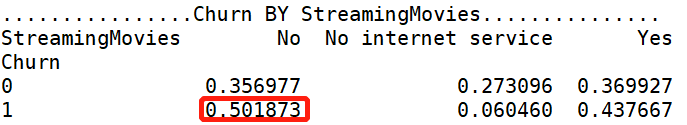【StreamingMovies 分析】：是否开通“网络电影”服务，对用户留存、流失，没有明显的促进作用。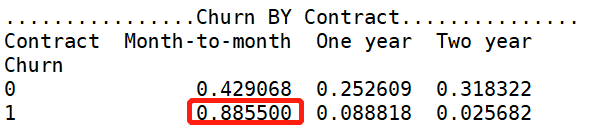【Contract 分析】逐月签订合同的用户最容易流失。### 8.2 均值比较

#### 8.2.0 齐性检验，方差分析

``#自定义齐性检验 & 方差分析 函数def ANOVA(x):    li_index=list(df['Churn'].value_counts().keys())    args=[]    for i in li_index:        args.append(df[df['Churn']==i][x])    w,p=stats.levene(*args)             #齐性检验    if p<0.05:        print('警告：Churn BY {}的P值为{:.2f}，小于0.05，表明齐性检验不通过，不可作方差分析'.format(x,p),'\n')    else:        f,p_value=stats.f_oneway(*args) #方差分析        print('Churn BY {} 的f值是{},p_value值是{}'.format(x,f,p_value),'\n')        if p_value<0.05:            print('Churn BY {}的均值有显著性差异，可进行均值比较'.format(x),'\n')        else:            print('Churn BY {}的均值无显著性差异，不可进行均值比较'.format(x),'\n')``

``print('MonthlyCharges、TotalCharges的齐性检验 和方差分析结果如下:','\n')ANOVA('MonthlyCharges')ANOVA('TotalCharges')``

【输出】：
MonthlyCharges、TotalCharges的齐性检验 和方差分析结果如下:

### 8.3 总结

• SeniorCitizen：青年人
• Partner ：单身
• Dependents ：无经济独立
• InternetService：开通了 “Fiber optic 光纤网络”
• OnlineSecurity：没开通“网络安全服务”
• OnlineBackup：没开通“在线备份业务”
• DeviceProtection：没开通通了“设备保护业务
• TechSupport：没开通“技术支持服务”
• Contract：“按月”签订合同方式
• PaperlessBilling：开通电子账单
• PaymentMethod：使用“电子支票”支付的人

## 9.实战教程-特征工程

### 9.1 提取特征

``churn_var=df.iloc[:,2:20]churn_var.drop("PhoneService",axis=1, inplace=True)churn_var.head()``

### 9.2 处理“量纲差异大”

“MonthlyCharges"、"TotalCharges"两个特征跟其他特征相比，量纲差异大。处理量纲差异大，有两种方法：

1. 标准化
2. 离散化

#### 9.2.1 标准化

``scaler = StandardScaler(copy=False)scaler.fit_transform(churn_var[['MonthlyCharges','TotalCharges']])  #fit_transform拟合数据churn_var[['MonthlyCharges','TotalCharges']]=scaler.transform(churn_var[['MonthlyCharges','TotalCharges']])  #transform标准化print(churn_var[['MonthlyCharges','TotalCharges']].head() )#查看拟合结果``

【输出】#### 9.2.2 特征离散化

1、处理'MonthlyCharges'：

``#查看'MonthlyCharges'列的4分位churn_var['MonthlyCharges'].describe() ``离散操作
18.25=<churn_var['MonthlyCharges']<=35.5,标记 “1”
35.5<churn_var['MonthlyCharges']<=70.35,标记 “2”
70.35<churn_var['MonthlyCharges']<=89.85,标记 “3”
89.85=<churn_varf['MonthlyCharges']<=118.75，标记“4”

``#用四分位数进行离散churn_var['MonthlyCharges']=pd.qcut(churn_var['MonthlyCharges'],4,labels=['1','2','3','4'])churn_var['MonthlyCharges'].head()``

``#查看'TotalCharges'列的4分位churn_var['TotalCharges'].describe()``

18=<churn_var['TotalCharges']<=402,标记 “1”
402<churn_var['TotalCharges']<=1397,标记 “2”
1397<churn_var['TotalCharges']<=3786,标记 “3”
3786<churn_var['TotalCharges']<=8684,标记 “4”

``#用四分位数进行离散 churn_var['TotalCharges']=pd.qcut(churn_var['TotalCharges'],4,labels=['1','2','3','4'])churn_var['TotalCharges'].head()``

【输出】### 9.3 分类数据转换成“整数编码”

#### 9.3.1 查看churn_var中分类变量的label(标签）

``#自定义函数获取分类变量中的labeldef Label(x):    print(x,"--" ,churn_var[x].unique()) #筛选出数据类型为“object”的数据点df_object=churn_var.select_dtypes(['object']) print(list(map(Label,df_object)))``

``churn_var.replace(to_replace='No internet service',value='No',inplace=True)``

``churn_var.replace(to_replace='No phone service',value='No',inplace=True)df_object=churn_var.select_dtypes(['object']) print(list(map(Label,df_object.columns)))``

#### 9.3.2 整数编码

1. sklearn中的LabelEncoder()
2. pandas中的factorize
此处选用 LabelEncoder()
``def labelencode(x):    churn_var[x] = LabelEncoder().fit_transform(churn_var[x])for i in range(0,len(df_object.columns)):    labelencode(df_object.columns[i])print(list(map(Label,df_object.columns)))``

### 9.4 处理“样本不均衡”

``x=churn_vary=df['Churn'].valuesprint('抽样前的数据特征',x.shape)print('抽样前的数据标签',y.shape)``

【输出】

1. 分层抽样
2. 过抽样
3. 欠抽样

``sss=StratifiedShuffleSplit(n_splits=5, test_size=0.2, random_state=0)print(sss)print("训练数据和测试数据被分成的组数：",sss.get_n_splits(x,y))# 分拆训练集和测试集for train_index, test_index in sss.split(x, y):    print("train:", train_index, "test:", test_index)    x_train,x_test=x.iloc[train_index], x.iloc[test_index]    y_train,y_test=y[train_index], y[test_index]``

“过抽样”让模型精度更高，故我选“过抽样”。

``from imblearn.over_sampling import SMOTEmodel_smote=SMOTE()x,y=model_smote.fit_sample(x,y)x=pd.DataFrame(x,columns=churn_var.columns)#分拆数据集：训练集 和 测试集x_train,x_test,y_train,y_test=train_test_split(x,y,test_size=0.3,random_state=0)``

``print('过抽样数据特征：', x.shape,      '训练数据特征：',x_train.shape,      '测试数据特征：',x_test.shape)print('过抽样后数据标签：', y.shape,      '   训练数据标签：',y_train.shape,      '   测试数据标签：',y_test.shape)``

【输出】

## 10.实战教程-数据建模

``Classifiers=[["Random Forest",RandomForestClassifier()],             ["Support Vector Machine",SVC()],             ["LogisticRegression",LogisticRegression()],             ["KNN",KNeighborsClassifier(n_neighbors=5)],             ["Naive Bayes",GaussianNB()],             ["Decision Tree",DecisionTreeClassifier()],             ["AdaBoostClassifier", AdaBoostClassifier()],             ["GradientBoostingClassifier", GradientBoostingClassifier()],             ["XGB", XGBClassifier()],             ["CatBoost", CatBoostClassifier(logging_level='Silent')]  ]``

``Classify_result=[]names=[]prediction=[]for name,classifier in Classifiers:    classifier=classifier    classifier.fit(x_train,y_train)    y_pred=classifier.predict(x_test)    recall=recall_score(y_test,y_pred)    precision=precision_score(y_test,y_pred)    f1score = f1_score(y_test, y_pred)    class_eva=pd.DataFrame([recall,precision,f1score])    Classify_result.append(class_eva)    name=pd.Series(name)    names.append(name)    y_pred=pd.Series(y_pred)    prediction.append(y_pred)``

## 11.模型评估

``names=pd.DataFrame(names)names=names.tolist()result=pd.concat(Classify_result,axis=1)result.columns=namesresult.index=["recall","precision","f1score"]result``

【输出】

## 12.基于“XGB”模型输出特征重要性

• CatBoost算法
``model = CatBoostClassifier()model.fit(x_train,y_train,eval_set=(x_test, y_test),plot=True)#特征重要性可视化catboost=pd.DataFrame(columns=['feature','feature_importance'])catboost['feature']=model.feature_names_catboost['feature_importance']=model.feature_importances_catboost=catboost.sort_values('feature_importance',ascending=False) #降序排列plt.figure(figsize=(10,10))plt.title('特征重要性')sns.barplot(x='feature_importance',y='feature',data=catboost)``

【输出】-XGB 算法

``model_xgb= XGBClassifier()model_xgb.fit(x_train,y_train)from xgboost import plot_importanceplot_importance(model_xgb,height=0.5)plt.show()``

【输出】由于 XGB算法精度得分最高，故我们以XGB得到的“特征重要性”进行分析。
【分析】

1. 第一重要特征：tenure
``plt.figure(figsize=(20,4))sns.countplot(x='tenure',hue='Churn',data=df)``

【输出】【分析】

1. 第二重要特征：PaymentMethod

【分析】

1. 第三重要特征：MonthlyCharges
查看流失用户、留存用户在付费方面的偏好：
'MonthlyCharges'、'TotalCharges'，离散化后，可进行卡方检验，然后交叉分析。
• 卡方检验：'MonthlyCharges'、'TotalCharges'
``df['MonthlyCharges-']=churn_var['MonthlyCharges']df['TotalCharges-']=churn_var['TotalCharges']print('kf_var的卡方检验结果如下:','\n')KF('MonthlyCharges-')KF('TotalCharges-')``

【输出】
kf_var的卡方检验结果如下:

Churn by MonthlyCharges 的卡方临界值是0.00，小于0.05，表明MonthlyCharges组间有显著性差异,可进行【交叉分析】

Churn by TotalCharges 的卡方临界值是0.00，小于0.05，表明TotalCharges组间有显著性差异,可进行【交叉分析】

• 交叉分析
``for i in ['MonthlyCharges','TotalCharges']:    print('................Churn BY {}...............'.format(i))    print(pd.crosstab(df['Churn'],df[i],normalize=0),'\n')``

【输出】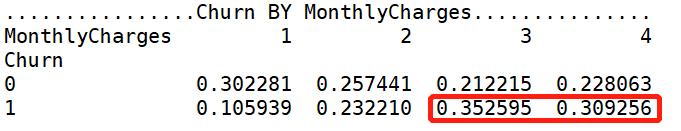18.25=<churn_var['MonthlyCharges']<=35.5,标记 “1”
35.5<churn_var['MonthlyCharges']<=70.35,标记 “2”
70.35<churn_var['MonthlyCharges']<=89.85,标记 “3”
89.85=<churn_varf['MonthlyCharges']<=118.75，标记“4”
【分析】

402<churn_var['TotalCharges']<=1397,标记 “2”
1397<churn_var['TotalCharges']<=3786,标记 “3”
3786<churn_var['TotalCharges']<=8684,标记 “4”
【分析】

`` print('MonthlyCharges的均值是{:.2f},TotalCharges的均值是{:.2f}'.format(df['MonthlyCharges'].mean(),df['TotalCharges'].mean()))``

``df_1=df[df['Churn']==1] #流失客户df_0=df[df['Churn']==0] #留存客户plt.figure(figsize=(10,10))   sns.scatterplot('MonthlyCharges','TotalCharges',hue='Churn', palette=plt.cm.RdYlBu,data=df_1)plt.axhline(y=df['TotalCharges'].mean(),ls="-",c="k")plt.axvline(x=df['MonthlyCharges'].mean(),ls="-",c="green")``

【输出】【分析】

``plt.figure(figsize=(10,10)) sns.scatterplot('MonthlyCharges','TotalCharges',hue='Churn', palette=plt.cm.RdYlBu_r,data=df_0)plt.axhline(y=df['TotalCharges'].mean(),ls="-",c="k")plt.axvline(x=df['MonthlyCharges'].mean(),ls="-",c="green")``

【输出】【结论】

1. tenure：1-5号职位的用户比较容易流失
2. PaymentMethod：使用“电子支票”支付的人
3. MonthlyCharges 、TotalCharges:总费用在2281.92元以下，月费用在64.76元以上的客户比较容易流失
4. PaperlessBilling:开通电子账单
5. Partner:单身
6. OnlineBackup:没开通“在线备份业务”
7. InternetService：开通了 “Fiber optic 光纤网络”
8. TechSupport：没开通“技术支持服务”
9. DeviceProtection：没开通通了“设备保护业务
10. OnlineSecurity：没开通“网络安全服务”
11. Contract：“按月”签订合同方式
12. Dependents：无经济独立
13. SeniorCitizen ：青年人
14. TotalCharges：总费用在2281.92元以下，月费用在64.76元以上的客户比较容易流失

​Python实用宝典 ( pythondict.com )